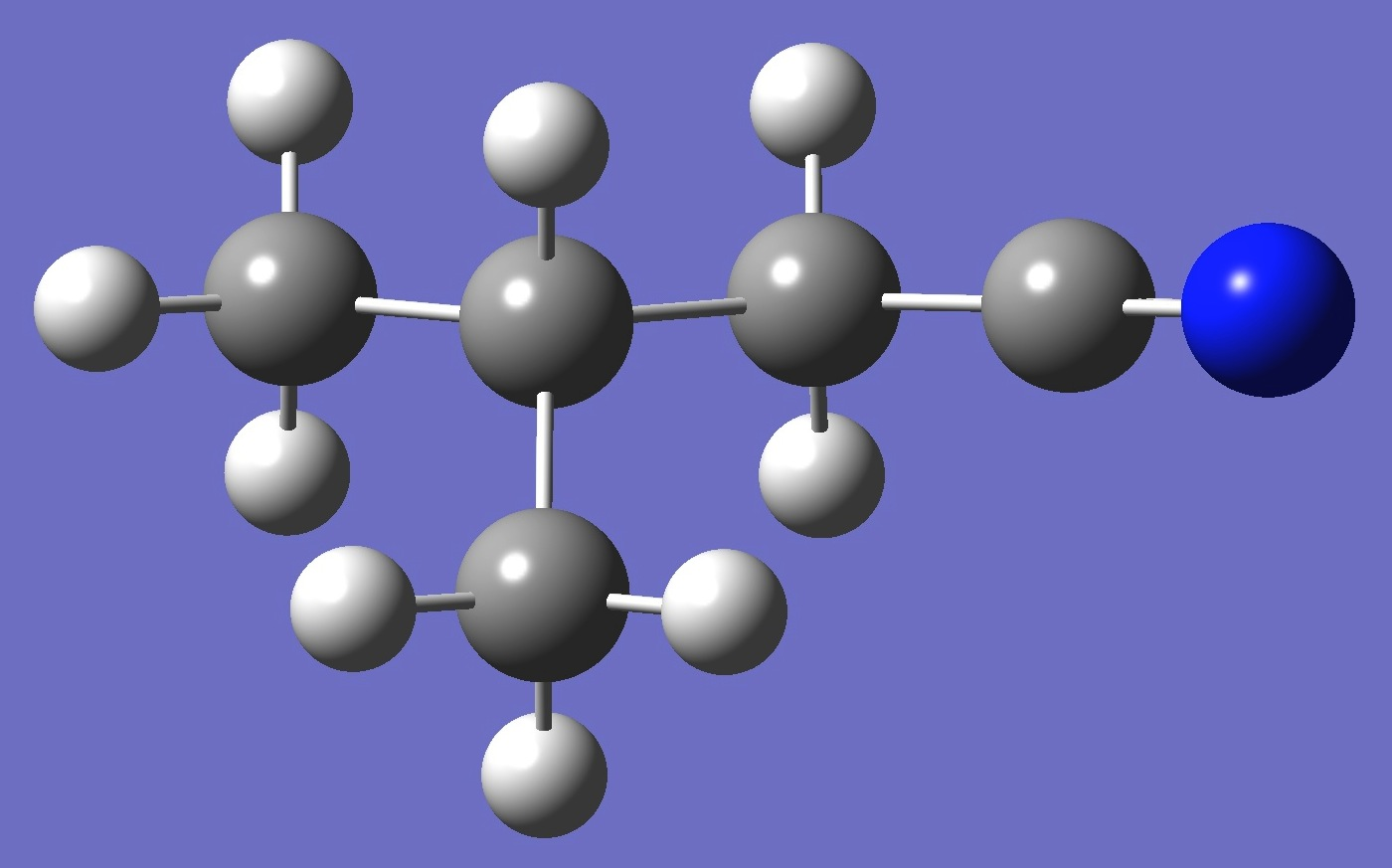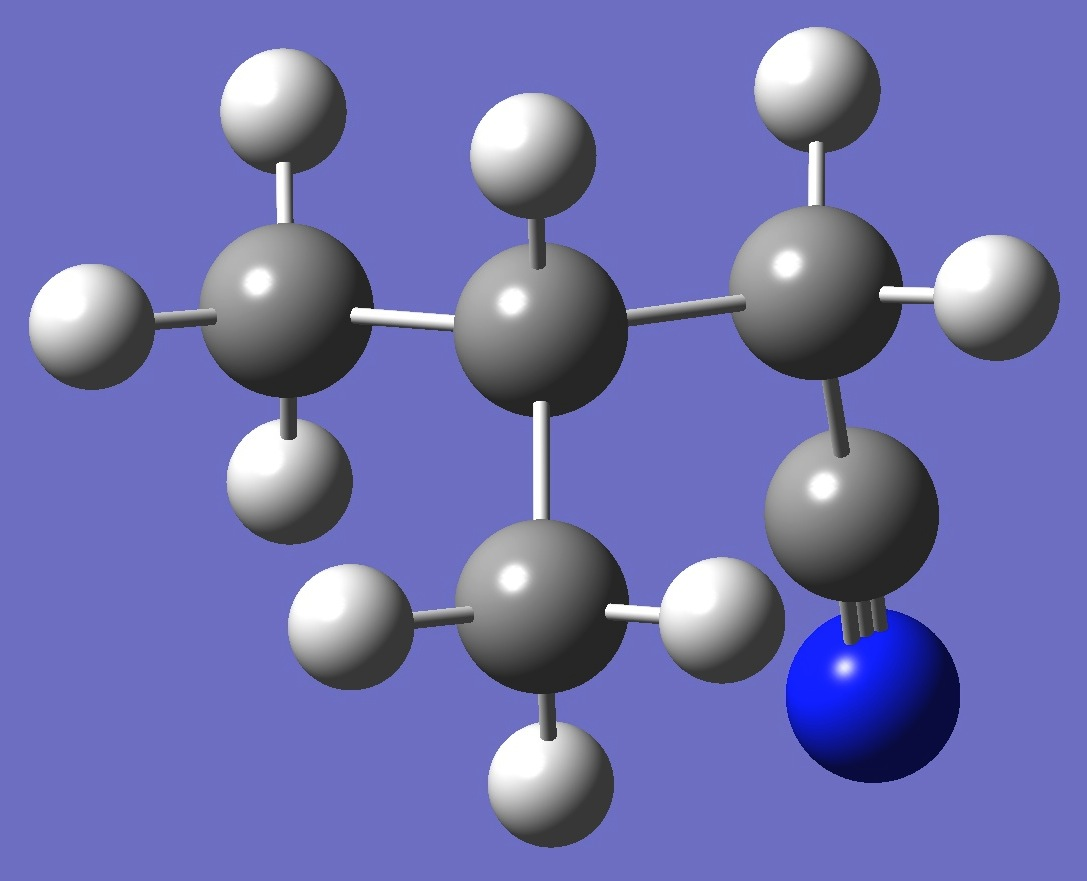C5H9N

Nitrogen

Nuclear Quadrupole Coupling Constants

in 3-Methylbutyronitrile

Nitrogen nqcc'c in anti and gauche conformers of 3-methylbutyronitrile shown below were determined by Wehres et al. .

anti

gaucheCalculations of the nitrogen nqcc tensors were made on an approximate equilibrium structures (~re) given by MP2/6-311+G(3df,3pd) optimization with empirically corrected CC and CN bond lengths.   These calculated nqcc's are compared with the experimental results in Tables 1 and 2.  Structure parameters are given in Table 3, rotational constants in Table 4.

In Tables 1 and 2, subscripts a,b,c refer to the principal axes of the inertia tensor; x,y,z to the principal axes of the nqcc tensor.  ETA = (Xxx - Xyy)/Xzz.  Ø (degrees) is the angle between its subscripted parameters.

RMS is the root mean square difference between calculated and experimental diagonal nqcc's (percentage of the average of the magnitudes of the experimental nqcc's).  RSD is the calibration residual standard deviation for the B3PW91/6-311+G(df,pd) model for calculation of the nitrogen  efg's/nqcc's.

 Table 1.  Nitrogen nqcc's in anti 3-Methylbutyronitrile (MHz).  Calculation was made on ~re molecular strcuture. Calc Expt.  14N Xaa - 3.050 - 3.07713(67) Xbb 1.214 1.24843(87) Xcc 1.836 1.82870(66) Xab - 2.163 Xac 1.151 Xbc 0.420 RMS 0.025 (1.2 %) RSD 0.030 (1.3 %) Xxx 2.043 Xyy 2.160 Xzz - 4.203 ETA 0.0278 Øz,CN

 Table 2.  Nitrogen nqcc's in gauche 3-Methylbutyronitrile (MHz).  Calculation was made on ~re molecular strcuture. Calc Expt.  14N Xaa - 1.378 - 1.44002(59) Xbb 2.015 2.01104(76) Xcc - 0.637 - 0.57102(66) Xac - 3.174 RMS 0.052 (3.9 %) RSD 0.030 (1.3 %) Xxx 2.015 Xyy 2.188 Xzz - 4.203 ETA 0.0412 Øz,CN

 Table 3.  3-Methylbutyronitrile: MP2/6-311+G(3df,3pd) optimized structure parameters, with approximate equilibrium bond lengths (~re) given in parentheses. anti gauche C  C,1,B1  H,2,B2,1,A1  H,1,B3,2,A2,3,D1,0  H,1,B4,2,A3,3,D2,0  H,1,B5,2,A4,3,D3,0  C,2,B6,1,A5,5,D4,0  C,2,B7,1,A6,7,D5,0  H,7,B8,2,A7,1,D6,0  H,7,B9,2,A8,1,D7,0  H,7,B10,2,A9,1,D8,0  H,8,B11,2,A10,1,D9,0  H,8,B12,2,A11,1,D10,0  C,8,B13,2,A12,1,D11,0  N,14,B14,2,A13,1,D12,0 C  C,1,B1  H,2,B2,1,A1  H,1,B3,2,A2,3,D1,0  H,1,B4,2,A3,3,D2,0  H,1,B5,2,A4,3,D3,0  C,2,B6,1,A5,5,D4,0  C,2,B7,1,A6,7,D5,0  H,7,B8,2,A7,1,D6,0  H,7,B9,2,A8,1,D7,0  H,7,B10,2,A9,1,D8,0  H,8,B11,2,A10,1,D9,0  H,8,B12,2,A11,1,D10,0  C,8,B13,2,A12,1,D11,0  N,14,B14,8,A13,2,D12,0 B1=1.52242121     (1.5203)  B2=1.09266218  B3=1.08934663  B4=1.08921845  B5=1.09173382  B6=1.52294086     (1.5206)  B7=1.53492397     (1.5322)  B8=1.08991737  B9=1.09142207  B10=1.089111  B11=1.09235812  B12=1.09083247  B13=1.45934342   (1.4602)  B14=1.16982551   (1.1564)  A1=108.92169314  A2=111.55375803  A3=110.56620483  A4=110.19950287  A5=111.15152338  A6=110.84308845  A7=111.56177437  A8=110.31987051  A9=110.66657166  A10=109.54887994  A11=110.45287722  A12=112.12652579  A13=143.22730256  D1=-59.30943958  D2=61.15229745  D3=-179.57031831  D4=-59.01154317  D5=-121.50788792  D6=179.26325682  D7=-60.48332502  D8=58.81600963  D9=60.37108753  D10=178.60271574  D11=-60.24503313  D12=-54.46119695 B1=1.52283823     (1.5207)  B2=1.09274994  B3=1.0901029  B4=1.08919971  B5=1.09082136  B6=1.52283823     (1.5207)  B7=1.53683957     (1.5340)  B8=1.0901029  B9=1.09082136  B10=1.08919971  B11=1.0909123  B12=1.0909123  B13=1.46086913    (1.4617)  B14=1.16995947    (1.1565)  A1=108.88883651  A2=111.44533917  A3=110.53863322  A4=110.40465686  A5=111.17652094  A6=110.84384673  A7=111.44533917  A8=110.40465686  A9=110.53863322  A10=110.41847089  A11=110.41847089  A12=111.53415009  A13=178.0510418  D1=-59.3265669  D2=60.98404473  D3=-179.58139441  D4=-58.98227523  D5=-123.76672187  D6=179.29288686  D7=-60.45228563  D8=58.98227523  D9=-177.51575622  D10=-58.52710727  D11=61.97856825  D12=0.

 Table 4.  3-Methylbutyronitrile:  Rotational Constants (MHz). ~re Expt.  anti A 7470. 7363.745484(92) B 2136. 2107.1641996(219) C 1787. 1762.0787378(211) gauche A 5324. 5293.815739(54) B 2504. 2456.4351918(226) C 2324. 2270.2489771(220)

tert Butyl Cyanide
2-Methylbutyronitrile n-Butyl Cyanide

 N.Wehres, M.Hermanns, O.H.Wilkins, K.Borisov, F.Lewen, J.-U.Grabow, S.Schlemmer, H.S.P.Müller, A&A 615,A140(2018).

N.Wehres, M.Hermanns, O.H.Wilkins, K.Borisov, F.Lewen, J.-U.Grabow, S.Schlemmer, H.S.P.Müller, Abstract WJ04, 72rd ISMS, Champaign-Urbana, Illinois, 2018.

Table of Contents

Molecules/Nitrogen

3MeBuCN.html

Last Modified 28 April 2018